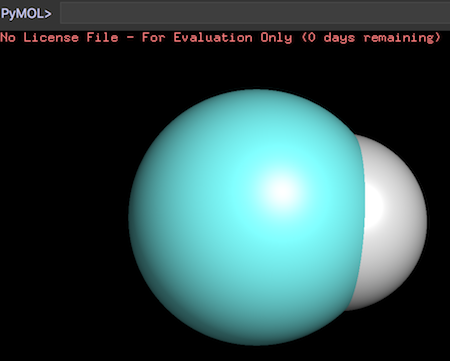"get_area" - Surface Areas of Atoms

This section provides a tutorial on how to use the 'get_area' command to measure surface areas of atoms.

PyMol allows you to measure surface areas of the atoms, using the "get_area" command.

For example, the following commands print areas of the carbon atom a hydrogen atom of the methane molecule:

```PyMOL># load the carbon atom
PyMOL>load Carbon-Atom.sdf

PyMOL># add 4 hydrogen atoms to create methane
PyMOL>h_add

PyMOL># print surface area of the carbon atom
PyMOL>get_area id 1
cmd.get_area: 15.171 Angstroms^2.

PyMOL># print surface area of a hydrogen atom
PyMOL>get_area id 2
cmd.get_area: 8.258 Angstroms^2.
```

Based the above output, we can calculate the radius of each atom, using the sphere surface area formula: area = 4 * PI * radius * radius.

```PyMOL>import math

PyMOL>print str( math.sqrt(15.171/(4*3.14159)) )
1.098758772689268

PyMOL>print str( math.sqrt(8.258/(4*3.14159)) )
0.8106487001768432
```

Now we can calculate the distance between these 2 atoms. Then we can compare the distance with their radiuses and see how much they overlap with each other:

```PyMOL>get_distance id 1, id 2
cmd.get_distance: 1.090 Angstroms.
```

If our calculation is correct, half of the hydrogen atom is overlapped with the carbon atom. This can be verified by displaying atoms as spheres.

```PyMOL>show spheres, id 1

PyMOL>show spheres, id 2
```Surface Areas of Atoms in PyMol

Table of Contents